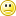## 2048 Minimum Winning Score

The too addictive2048 puzzle is a 4x4 grid of number tiles: (2, 4, 8, 16, ... up to 2048 ... and perhaps 131072). When two tiles of the same value are "slid together" they merge into one tile with twice the value, and the score is incremented by the value of the merged tile. For most people playing the game, the goal is the maximum score, somewhere in the millions, usually with an "undo" (then redo) version of the game, and many days of effort.

But what is the minimum winning score? Naively, it would be a series of ALL starting 4 tiles merged perfectly together into a single 2048 tile, with no leftovers larger than 4. 512 4-tiles merge to 256 8-tiles: added score 2048 points. 256 8-tiles merge to 16-tiles, 2048 more points. In 7 more perfect merge steps ( 32, 64, 128, 256, 512, 1024, 2048 ) reaches a score of 9*2048, 18432 points.

However, in reality, approximately 90% of the starting tiles are 2's, which add more points when they merge into 4s. How many more points is that? We still "pass through" 512 4's, but most are from 2 merges. What fraction best case, what fraction on average, what fraction an "achievable" case?

The original 2048 code uses a 90% random probability of spawning a 2-tile, and a 10% probability of spawning a 4-tile. If we spawn 20 tiles, that would be 18 2-tiles and 2 4-tiles.

Assume all 18 2-tiles merge into 9 4-tiles; that generates 36 points. The original 4 tiles generate no points. The average result is 11 4-tiles and 36 added points, hence the average points for a 4-tile is 36/11 points or 3.2727... points. We need 512 4-tiles, so the average number of points created by generating 512-4 tiles is 512*36/11 or 1675.6363... points. Added to 18432 points for the next 9 merges, the resulting average score (assuming no left-over merged tiles besides the 2048 tile) is a hair less than 20108 points.

20108 points is annoyingly close to 19999 points ... and it is the result of a random process which will randomly deviate (plus or minus a sizable variance) from the average 20108 points.

How much variation? That gets a bit complicated; the statistics can be described by a "2/11 weighted" binomial distribution.

The binomial distribution formula involves some ENORMOUS multipliers, such as 256 factorial. Fortunately, there's a trick. The number is a large number of multiplied and divided factors, which can all be converted to logarithms and added rather than multiplied. 256 factorial is a 507 digit decimal integer; but ln(256!) is a floating point number less than 600.

Here's a table of results. Yes, there are too many useless decimal places, but useful for finding computation errors.

The probability of an "all 4-tile" game is less than 1e-400, which is zero on my 64 bit Pentium CPU. Don't hold your breath waiting for that to happen.

I will add the code and the graph later. My coding skills are embarrassing, and the results need better checking.

The binomial distribution graph peaks at 419 pairs of 2-tiles (1676 merge points, 20108 total points ) with a cumulative probability near 0.5; to shed 108 points from that means 28 or more 4 tiles, with a cumulative probability of 8e-4 . If there were no left over merged tiles, that suggests a 50% chance of a "19996 or smaller score" after a "mere" 620 games.

But then there are those pesky extra merge tiles. The best I can usually do is around "40 points" of merge tiles.

The distribution is VERY steep at "4-tile" end of the distribution. To reduce by 40 more points (10 more starting 4 tiles) results in a cumulative probability of 1.25e-5, 64 times as many games, perhaps 40,000 games for a 50% chance of success.

I imagine a well-designed algorithm could reduce the number of left-over tiles somewhat. A software robot player might use this hypothetical algorithm against tens of thousands of simulated "Cirulli standard" 2048 games in less than an hour (WAG). I will leave the "20K-bot" project for a better programmer than I am; especially adding the graphics to illustrate the winning minimum game.

Note that the "Cirulli standard" game generates 9 2-tiles per 4-tile. A nonstandard game generating 8 or less 2-tiles per 4-tile would generate a "minimum win" MUCH faster. A very simple code tweak to the standard game, useful for testing the rest of the code.

Cheesy inept-programmer C program source: bin2048b

### Some example games, with estimated cost of surplus tiles20096-10.png20096-24.png20100-13.png20100-24.png20124-28.png20124-28-b.png20128-28.png20136-31.png20148-18.png20152-21.png20152-91.png20152-116.png20172-89.png20200-116.png

2048min (last edited 2023-08-30 23:09:26 by KeithLofstrom)